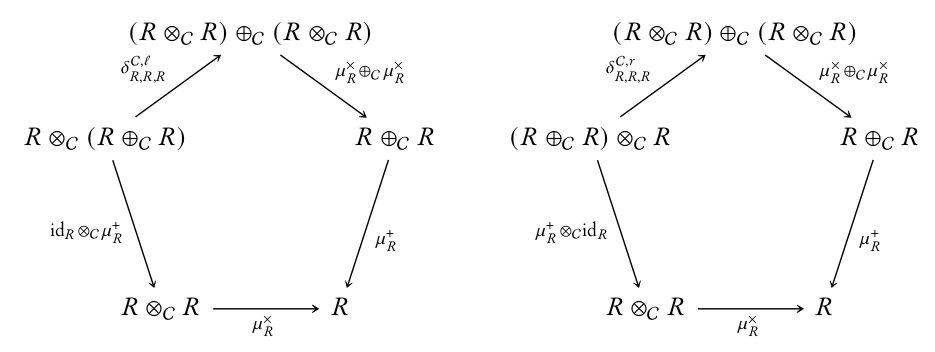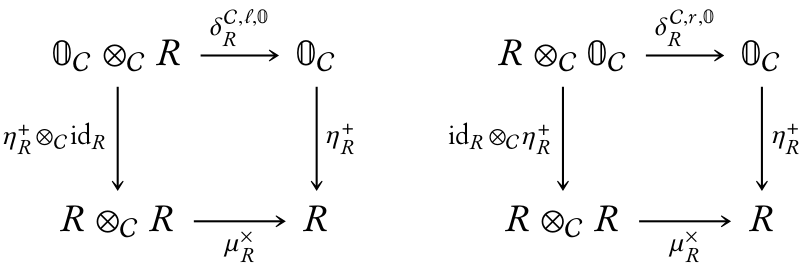# nLab ring object

Contents

### Context

#### Categorical algebra

internalization and categorical algebra

universal algebra

categorical semantics

# Contents

## Idea

For $C$ a cartesian monoidal category (a category with finite products), an internal ring or a ring object in $C$ is an internalization to the category $C$ of the notion of a ring.

Under some reasonable assumptions on $C$ that allow one to construct a (symmetric) monoidal tensor product on the category of abelian group objects $Ab(C)$ internal to $C$, a ring object can also be defined as a monoid object internal to that monoidal category $Ab(C)$.

Sometimes one might take this last point of view a little further, especially in certain contexts of stable homotopy theory where a stable (∞,1)-category of spectra is already something like an (∞,1)-category-analogue of a category of abelian groups. With the understanding that a symmetric smash product of spectra plays a role analogous to tensor products of abelian groups, monoids with respect to the smash product are often referred to as “$xyz$-rings” of one sort or another (as mentioned at “ring operad”). Thus we have carry-over phrases from the early days of stable homotopy theory, such as “A-∞ rings” (for monoids) and “E-∞ rings” (commutative monoids). Here it is understood that the monoid multiplication on spectra is an $(\infty, 1)$-refinement of a multiplicative structure on a corresponding cohomology theory, with various forms of K-theory providing archetypal examples.

## Definition

### As a model of a Lawvere theory

Let $T$ be the Lawvere theory for rings, viz. the category opposite to the category of finitely generated free rings (which are non-commutative polynomial rings $\mathbb{Z}\langle X_1, \ldots, X_n\rangle$) and ring maps between them. Then for $C$ a category with finite products, a ring object in $C$ may be identified with a product-preserving functor $T \to C$.

The more traditional definition, based on a traditional presentation of the equational theory? of rings, is that a ring object consists of an object $R$ in $C$ together with morphisms $a: R \times R \to R$ (addition), $m: R \times R \to R$ (multiplication), $0: 1 \to R$ (zero), $e: 1 \to R$ (multiplicative identity), $-: R \to R$ (additive inversion), subject to commutative diagrams in $C$ that express the usual ring axioms.

### Via the microcosm principle

Alternatively, one may define ring objects following the Baez–Dolan microcosm principle. Indeed, similarly to how it is possible to define monoids in a monoidal category (a pseudomonoid in $(\mathsf{Cat},\times,\mathsf{pt})$), it is possible to speak of semiring objects internal to any bimonoidal category (a pseudomonoid in $(\mathsf{SymMonCats},\otimes_{\mathbb{F}},\mathbb{F})$).

Namely, a semiring in a bimonoidal category $(\mathcal{C},\otimes_{\mathcal{C}},\oplus_{\mathcal{C}},\mathbf{0}_{\mathcal{C}},\mathbf{1}_{\mathcal{C}})$ is given by a quintuple $(R,\mu^{+}_{R},\eta^{+}_{R},\mu^{\times}_{R},\eta^{\times}_{R})$ consisting of

• An object $R$ of $\mathcal{C}$, called the underlying object of the semiring;
• A morphism
$\mu^{+}_{R}\colon R\oplus_{\mathcal{C}}R\longrightarrow R$

of $\mathcal{C}$, called the addition morphism of $R$;

• A morphism
$\mu^{\times}_{R}\colon R\otimes_{\mathcal{C}}R\longrightarrow R$

of $\mathcal{C}$, called the multiplication morphism of $R$;

• A morphism
$\eta^{+}_{R}\colon\mathbf{0}_{\mathcal{C}}\longrightarrow R$

of $\mathcal{C}$, called the additive unit morphism of $R$;

• A morphism
$\eta^{\times}_{R}\colon\mathbf{1}_{\mathcal{C}}\longrightarrow R$

of $\mathcal{C}$, called the multiplicative unit morphism of $R$;

satisfying the following conditions:

1. The triple $(R,\mu^{+}_R,\eta^{+}_R)$ is a commutative monoid in $\mathcal{C}$;

2. The triple $(R,\mu^{\times}_R,\eta^{\times}_R)$ is a monoid in $\mathcal{C}$;

3. The diagramscorresponding to the semiring axioms $a(b+c)=a b+a c$ and $(a+b)c=a c+b c$ commute;

4. The diagramscorresponding to the semiring axioms $0a=0$ and $a0=0$ commute;

Moreover, for $\mathcal{C}$ a braided bimonoidal category, one defines a commutative semiring in $\mathcal{C}$ to be a semiring in $\mathcal{C}$ whose multiplicative monoid structure is commutative.

A partial version of this definition first appeared in (Brun 2006, Definition 5.1).

## Examples

For the notion of a semiring in a bimonoidal category defined via the microcosm principle, we have the following examples.

• A semiring in $\left(\mathsf{Sets},\coprod,\times,\emptyset,\times\right)$ is a monoid.
• A semiring in $\left(\mathsf{CMon},\oplus,\otimes_\mathbb{N},0,\mathbb{N}\right)$ is a semiring.
• A semiring in $\left(\mathsf{Ab}, \oplus,\otimes_\mathbb{Z},0,\mathbb{Z}\right)$ is a ring.
• A semiring in $\left(\mathsf{Mod}_R,\oplus,\otimes_R,0,R\right)$ is an associative algebra.
• A semiring in $\left(\mathsf{Cats},\coprod,\times,\emptyset_{\mathsf{cat}},\mathsf{pt}\right)$ is a strict monoidal category.